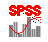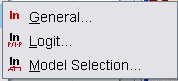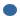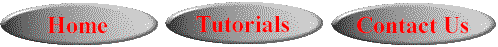# SPSS On-Line Training WorkshopHOME Table of Contents Data Editor Window Syntax  Editor WindowCarl Lee Felix Famoye About Us Chart Editor Window Output Window Overview of Data Analysis Manipulation of Data Analysis of Data Projects & Data Sets Integrate R into SPSS

# Log-Linear ModelsIn this Tutorial:

General
Log-linear Model

Analysis of DataLoglinear models include general loglinear model, logit  model and model selection techniques. The following movie clip demonstrates how to conduct a general log-linear model analysis.

In this on-line workshop, you will find many movie clips. Each movie clip will demonstrate some specific usage of SPSS.

General Loglinear Model:  General log-linear model is a technique for modeling a categorical response variable, which are often count data that follows a Poisson distribution or frequency in a cross tabulation form that follows a multinomial distribution, based on a set of factors or covariates. The maximum number of factors or covariates allowed is ten. The procedure analyzes the frequency counts of cases falling into each category of a cross classification of two or more variables. Each cross classification leads to a cell. The variables forming the cross classification are called factors. In this analysis, the frequency in each cell is the dependent variable. The dependent variable is assumed to follow a Poisson or multinomial distribution.

The assumptions required for count data following Poison distribution areThe event is statistically independent of cell counts in different cells.The variance of the response variable is the same as the mean of the count variable.

The assumptions required for the data following multinomial distribution are:The total sample size is fixed, that is the analysis is conditioned on the sample size.The cell counts are not independent from each other, since the count of a cell equals to the total sample size minus the counts of other cells.

Structural and Sampling Zero:

Suppose some of the cells in a cross classification contain structural zeros, then it is necessary to have a cell structure variable. A cell structure variable has a value of 0 or 1. Suppose a survey is sent out to find out how many times respondents went fishing last week. The survey was sent to people who fish and those who do not fish. For respondents who do not fish, the number of times is 0, which is a structural zero. For those who fish, but did not fish last week, the number of times is 0, which is a sampling zero.

Cell structure can also be used as in modeling accidents when data has been aggregated. The structure variable will be an offset.

The main dialog box has the following submenus:Save- To save some statistics like the residuals and predicted values.Model- To specify the model type. Here, you can select saturated or custom, where you specify the model terms.Options- To display some of the results in the analysis. One can request some plots and also specify the criteria for parameter estimation.

The data set for demonstrating regression modeling is the Tech Survey data set. See Data Set page for details. The purpose is to study the relationship between Q2: professor rank, like full professor or associate professor, and Q26: whether or not they use technology in the classroom, and to study if there is a significant relationship between Q2, Q26 and Q31A1 which deals with the level of difficulty when using technology.

Logit Loglinear Models are similar to ANOVA models for the logit-expected cell frequencies of crosstabulation tables.Dependent variables- These are categorical variables.Factors- These are categorical predictor variables which are used to form the cross classification.Covariates- These are scale predictors.Cell Structure- These variables allow you to exclude some cells from the analysis. These can be offset variable when a particular structure is required on the cross classification.Contrasts- These are variables to test the differences between model effects.

Logit Loglinear Analysis is used to analyze the relationship between categorical dependent variables and independent variables. The independent variables can be categorical (factors) or scale (covariates). One can have up to 10 dependent and factor variables combined. A cell structure variable allows one to define structural zeros or include an offset term in the model.

There are three submenus in the main dialog box:Save- To save some statistics like the residuals and predicted values.Model- To specify the model type. Here, you can select saturated or custom, where you specify the model terms.Options- To display some of the results in the analysis. One can request some plots and also specify the criteria for parameter estimation.

Model Selection Loglinear Analysis:

This is used to analyze multiway cross-tabulations. This analysis fits hierarchical loglinear models to cross-tabulations with many dimensions (factors). This will help to determine which categorical variables are related. Available methods are forced entry and backward elimination. It can be used to describe the relationship between categorical variables by analyzing the cell frequencies in a cross classification.

In this workshop, there is no movie clip to illustrate Logit Loglinear and Model Selection Loglinear Analyses.This online SPSS Training Workshop is developed by Dr Carl Lee, Dr Felix Famoye , student assistants Barbara Shelden and Albert Brown , Department of Mathematics, Central Michigan  University. All rights reserved.